• Introduction
• What is in this manual
• What is Caspoc
• User interface
• Introduction
• Starting
• Simulation
• Editing
• Viewing and printing
• Getting Started
• Basic editing
• Simulation in the time domain
• Basic User Interface Topics
• Editing
• Simulation
• Viewing
• Library
• Reports
• Project management
• Circuit and Block Diagram Components
• Introduction
• Cscript and user defined functions
• Component parameters
• Modeling Topics
• Introduction
• Power Electronics
• Semiconductors
• Electrical Machines
• Electrical drives
• Power Systems
• Mechanical Systems
• Thermal Systems
• Magnetic Circuits
• Green Energy
• Coupling to FEM
• Experimenter
• Analog hardware description language
• Embedded C code Export
• Coupling to Spice
• Small Signal Analysis
• Matlab coupling
• Tips and tricks
• Appendices

## Ideal semiconductor parameters.

The ideal semiconductor parameters are optimized for a high simulation speed and no convergence problems. You can set these parameters by using the ".Model" library. In the properties dialog box for a diode (Select it by right clicking the diode), you can specify the name of the ".Model". As default the model "Diode" is used for the ideal switch model of the diode.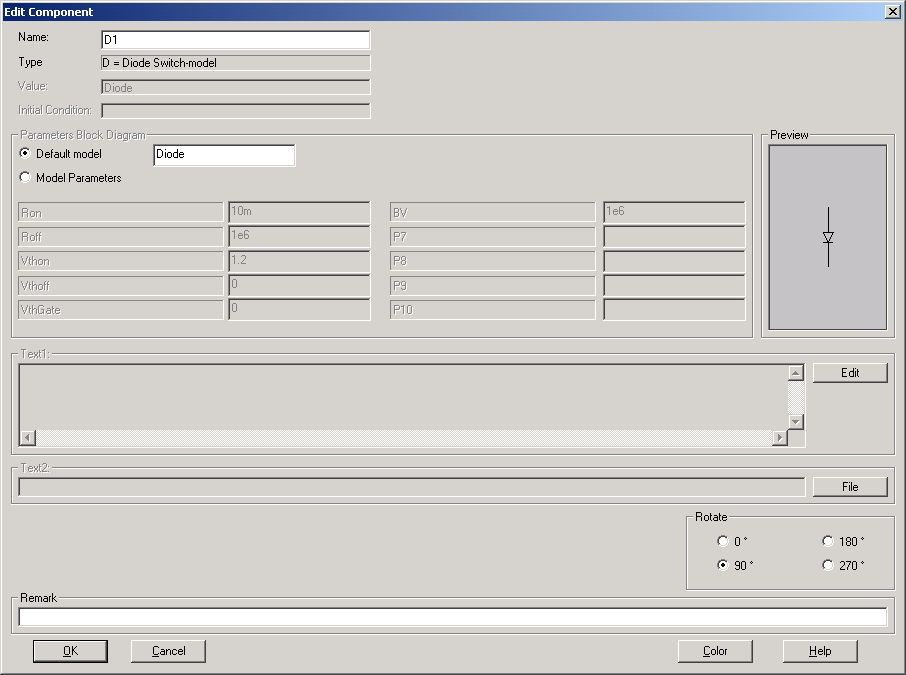Type here for example "MyModel"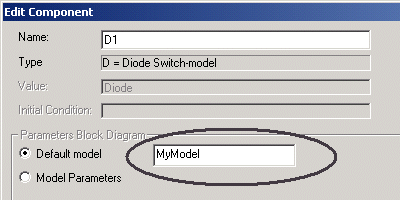Secondly you have to define your new "MyModel". Therefore you open the dialog box for the commands by selecting "Insert/Edit Commands". In the edit field, add the following line:

  .Model MyModel Dswitch Ron=10m VthOff=1 VthOn=1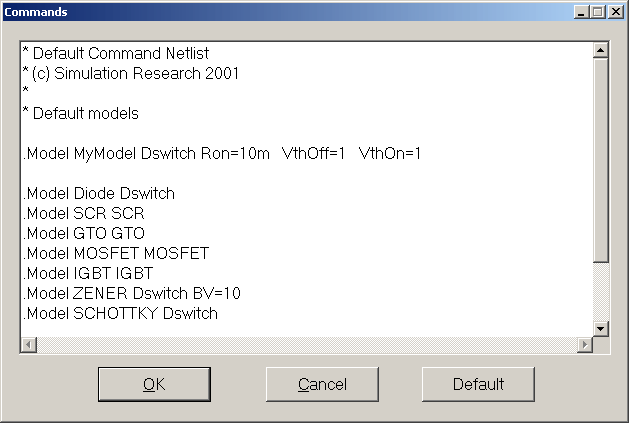This will create a model where the forward voltage drop over the diode equals 1 volt and the on resistance of the diode equals 10mOhm. The diode will turn on when the forward voltage is larger than VthOn and the diode will turn off as soon as the voltage over the diode is below VthOff.

All diodes where "MyModel" is specified at the edit field "Value", will have the forward voltage and on resistance as defined in the .Model description.

The following simulation shows the forward voltage and on resistance.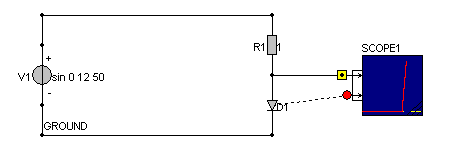When the current through the diode equals 10A, the voltage drop over the diode equals the voltage VthOff + 10*Ron = 1.1 volt.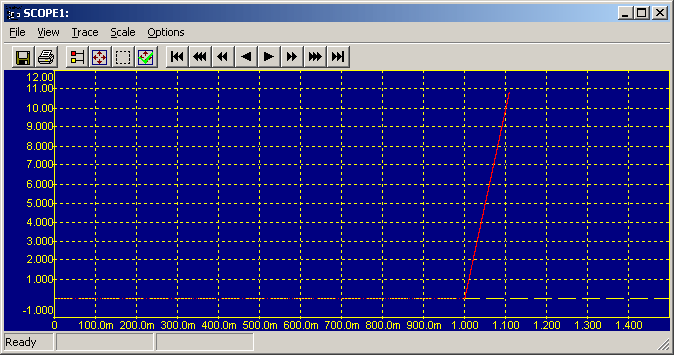You can also define the semiconductor parameters directly by selecting Model Parameters: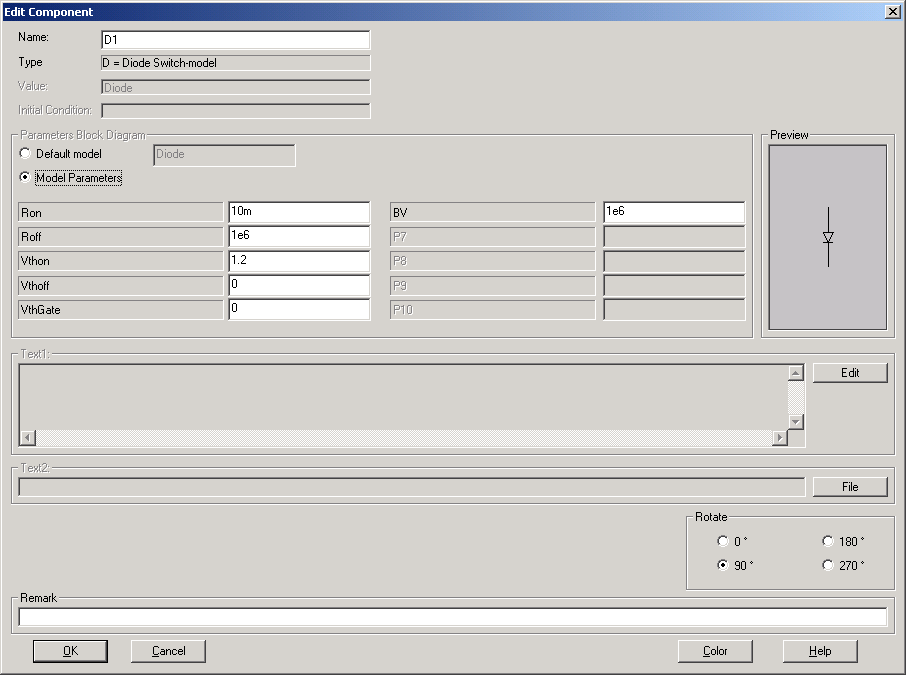Help on the parameters for the semiconductor can be found in the reference guide under .Model, or by selecting the [Help] button for the online help for the selected semiconductor.